# Map coloring: the color scale styles available in the tmap package

vignette
rstats
Author

Published

October 17, 2019

This vignette builds on the making maps chapter of the Geocomputation with R book. Its goal is to demonstrate all possible map styles available in the tmap package.

## Prerequisites

The examples below assume the following packages are attached:

``````library(spData) # example datasets
library(tmap)   # map creation
library(sf)     # spatial data reprojection``````

The `world` object containing a world map data from Natural Earth and information about countries’ names, regions, and subregions they belong to, areas, life expectancies, and populations. This object is in geographical coordinates using the WGS84 datum, however, for mapping purposes, the Mollweide projection is a better alternative (learn more in the modifying map projections section). The `st_tranform` function from the sf package allows for quick reprojection to the selected coordinate reference system (e.g., `"+proj=moll"` represents the Mollweide projection).

``world_moll = st_transform(world, crs = "+proj=moll")``

# One color

Let’s start with the basics. To create a simple world map, we need to specify the data object (`world_moll`) inside the `tm_shape()` function, and the way we want to visualize it. The tmap package offers several visualisation possibilities for polygons, including `tm_borders()`, `tm_fill()`, and `tm_polygons()`. The last one draws the filled polygons with borders, where the fill color can be specified with the `col` argument:

``````tm_shape(world_moll) +
tm_polygons(col = "lightblue")``````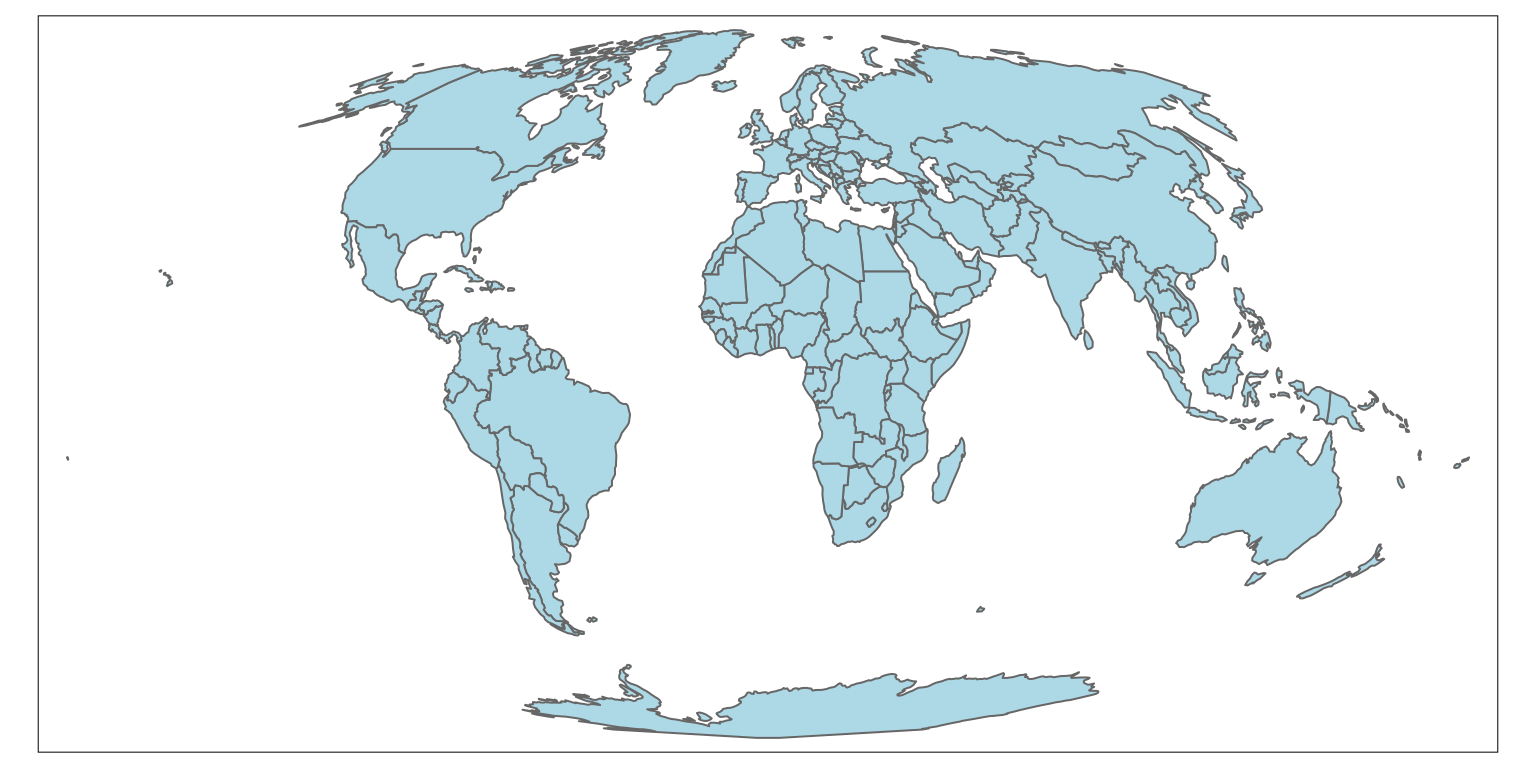The output is a map of world countries, where each country is filled with a light blue color.

The `col` argument is very flexible, and its action depends on the value provided. In the previous example, we provided a single color value resulting in a map with one color. To create a map, where adjacent polygons do not get the same color, we need to provide a keyword `"MAP_COLORS"`.

``````tm_shape(world_moll) +
tm_polygons(col = "MAP_COLORS")``````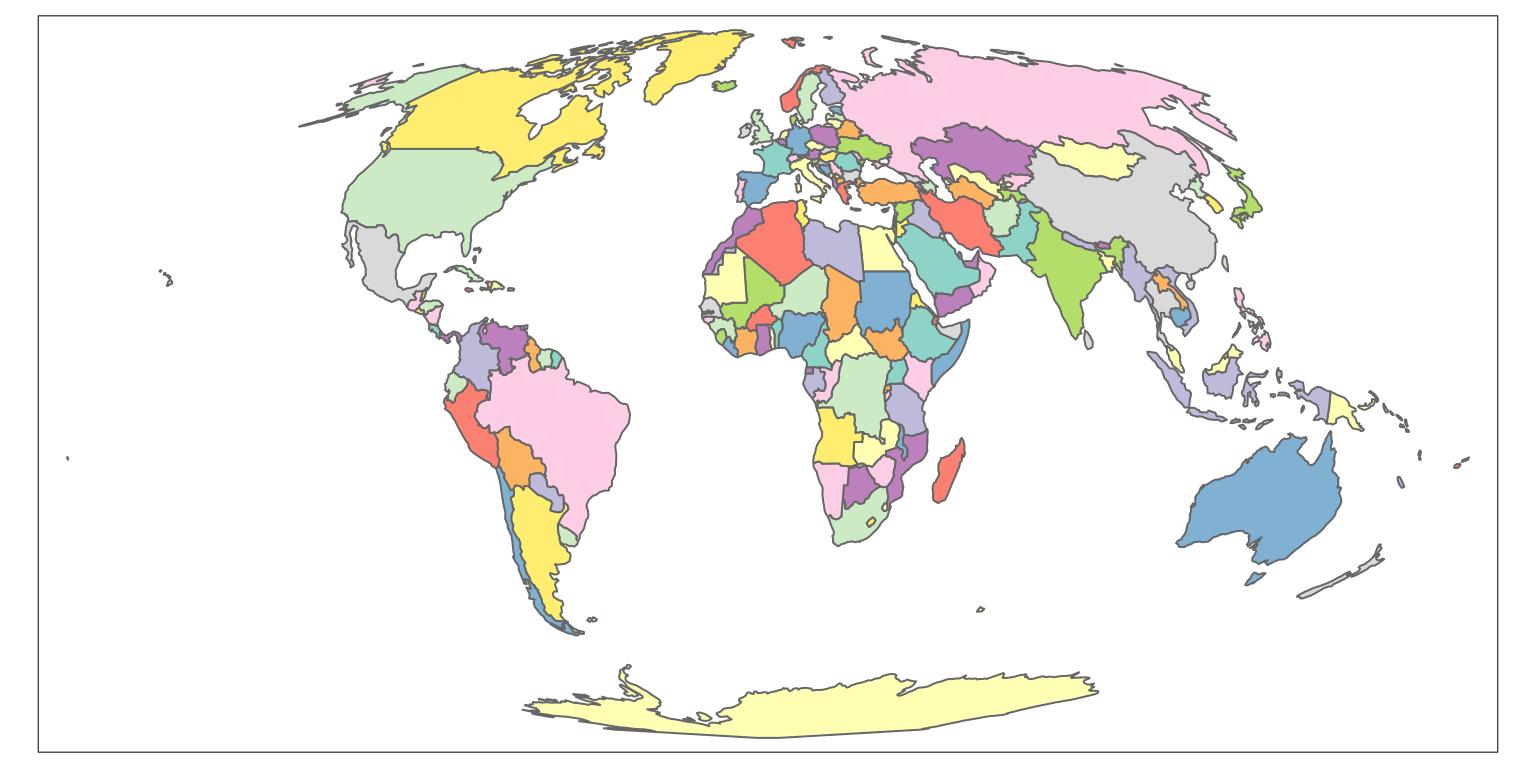The default color can be changed using the `palette` argument - run the `tmaptools::palette_explorer()` function to see possible palettes’ names.

``````tm_shape(world_moll) +
tm_polygons(col = "MAP_COLORS",
palette = "Pastel1")``````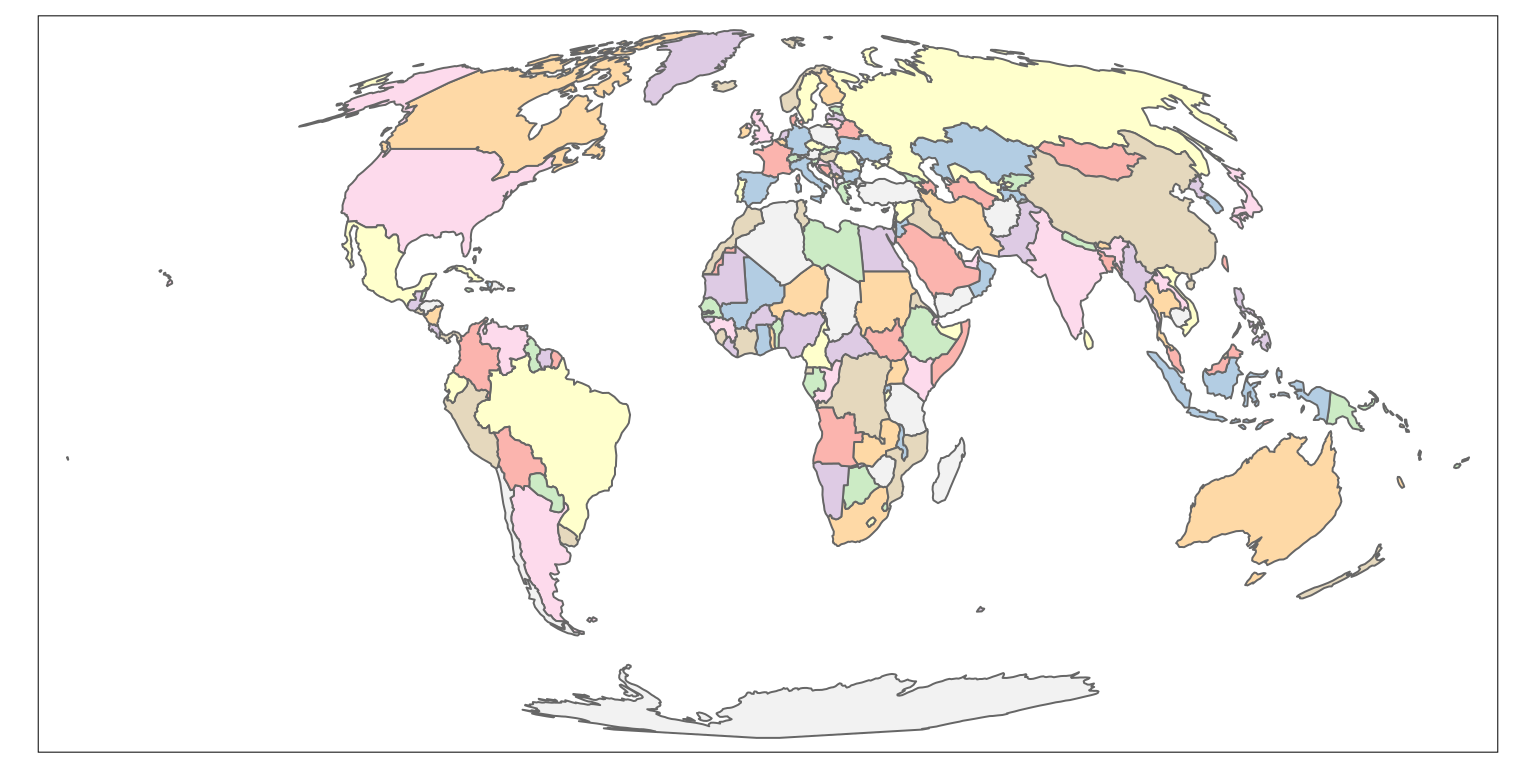Additionally, in this case, it is possible to use the `minimize` argument, which triggers the internal algorithm to search for a minimal number of colors for visualization.

``````tm_shape(world_moll) +
tm_polygons(col = "MAP_COLORS",
minimize = TRUE)``````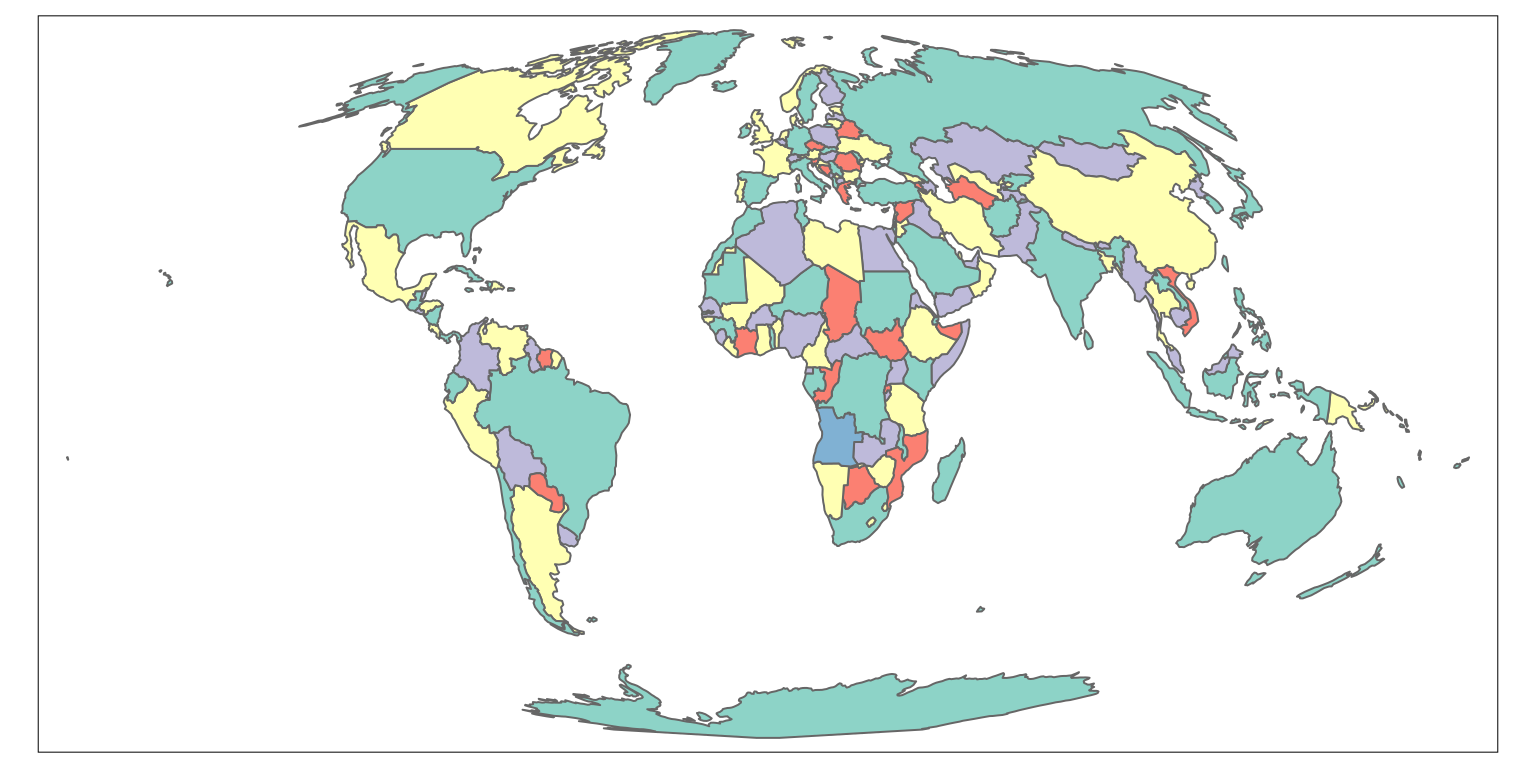The new map uses five colors. On a side note, in theory, no more than four colors are required to color the polygons of the map so that no two adjacent polygons have the same color (learn more about the four color map theorem on Wikipedia).

# Categorical maps

The third use of the `col` argument is by providing the variable (column) name. In this case, the map will represent the given variable. By default, tmap behaves differently depending on the input variable type. For example, it will create a categorical map when the provided variable contains characters or factors. The `tm_polygons(col = "subregion", style = "cat")` code will be run automatically in this case.

``````tm_shape(world_moll) +
tm_polygons(col = "subregion")+
tm_layout(legend.outside = TRUE) ``````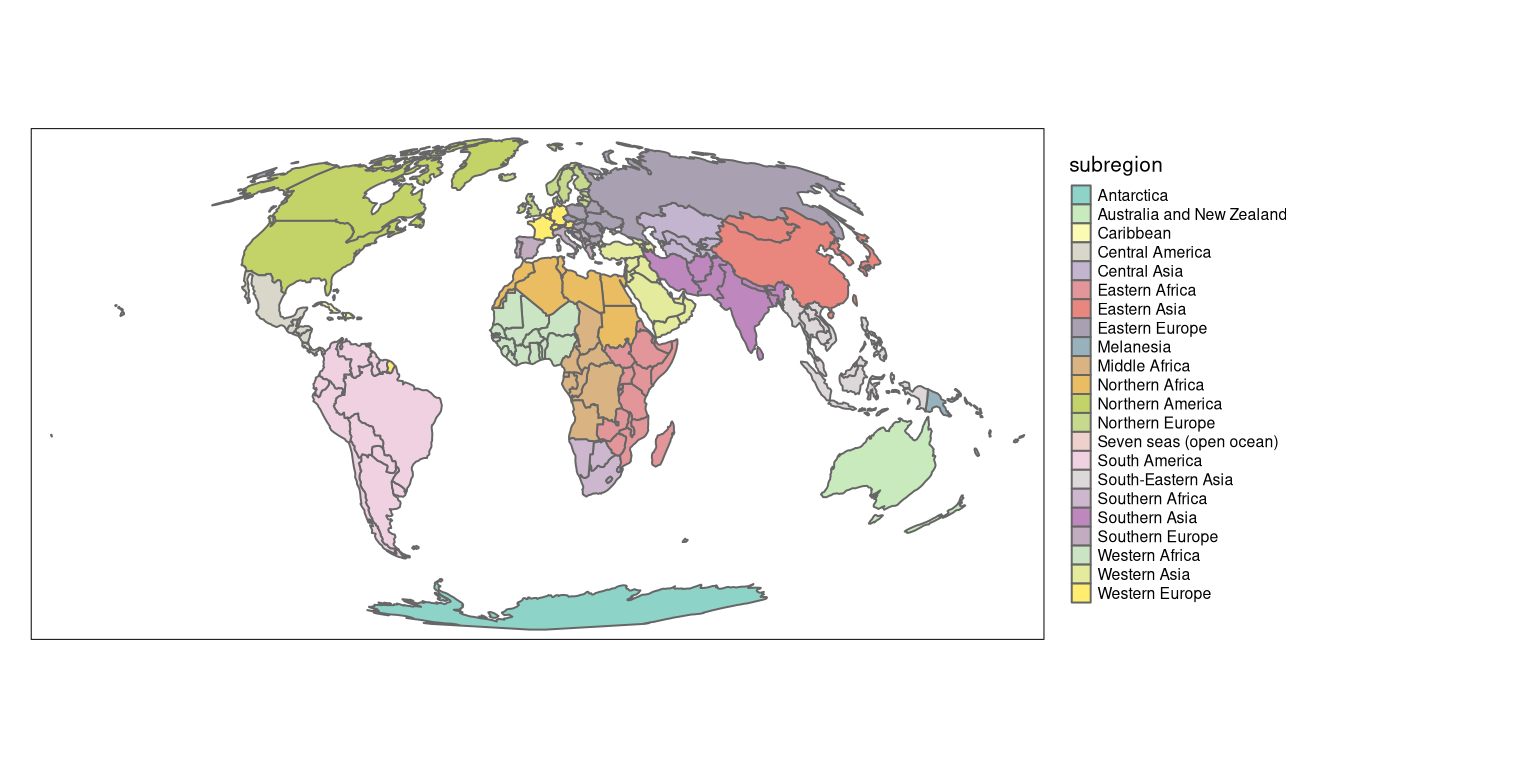# Discrete maps

Discrete maps represents continuous numerical variables using discrete class intervals. There are several ways to convert continuous variables to discrete ones implemented in tmap.

## Pretty

When the variable provided as the `col` argument is numeric, tmap will use the `"pretty"` style as a default. In other words, it runs `tm_polygons(col = "lifeExp", style = "pretty")` invisibly to the user. This style rounds breaks into whole numbers where possible and spaces them evenly.

``````tm_shape(world_moll) +
tm_polygons(col = "lifeExp",
legend.hist = TRUE) +
tm_layout(legend.outside = TRUE) ``````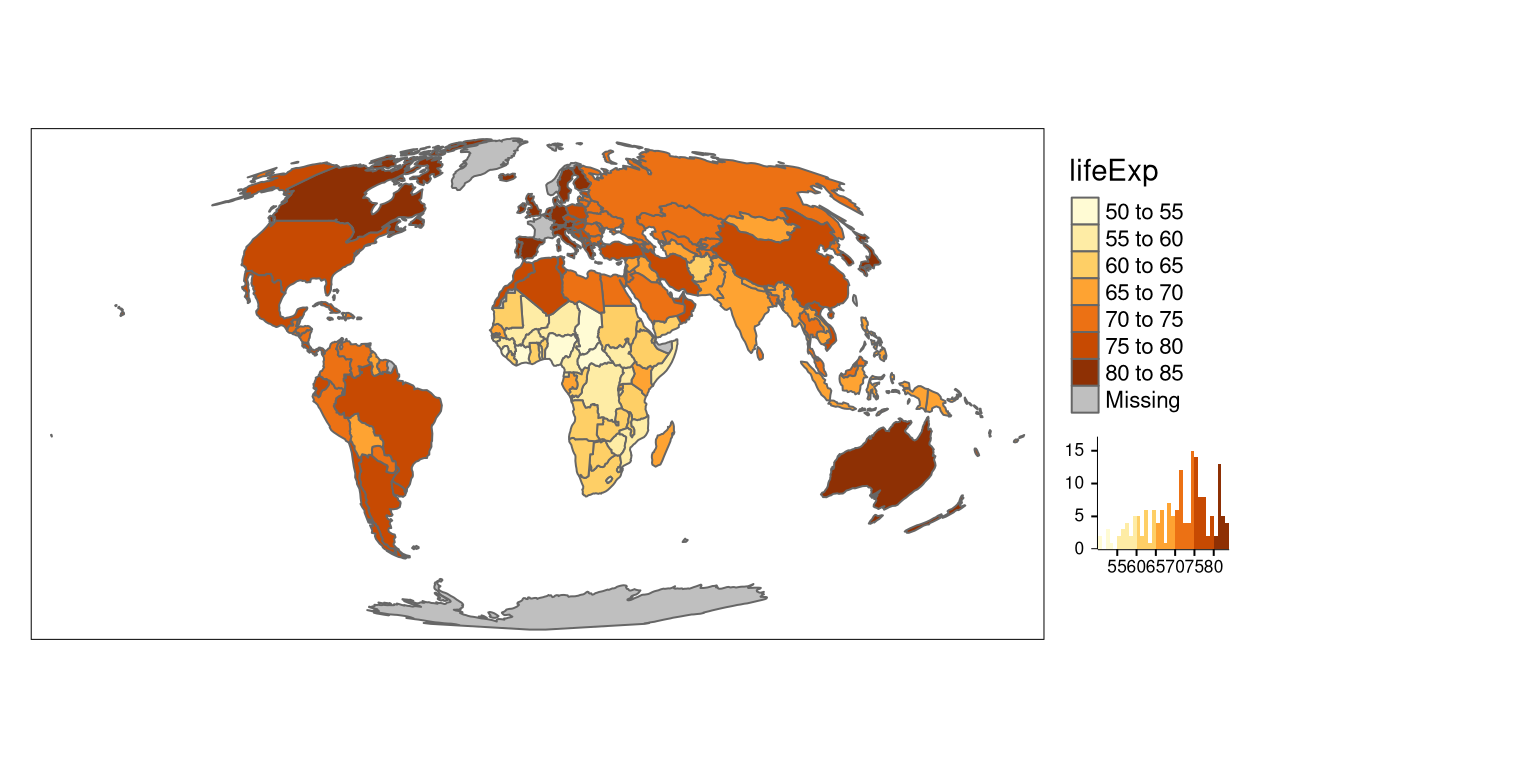A histogram is added using `legend.hist = TRUE` in this and several next examples to show how the selected map style relates to the distribution of values.

It is possible to indicate a preferred number of classes using the `n` argument. Importantly, not every `n` is possible depending on the range of the values in the data.

``````tm_shape(world_moll) +
tm_polygons(col = "lifeExp",
legend.hist = TRUE,
n = 4) +
tm_layout(legend.outside = TRUE) ``````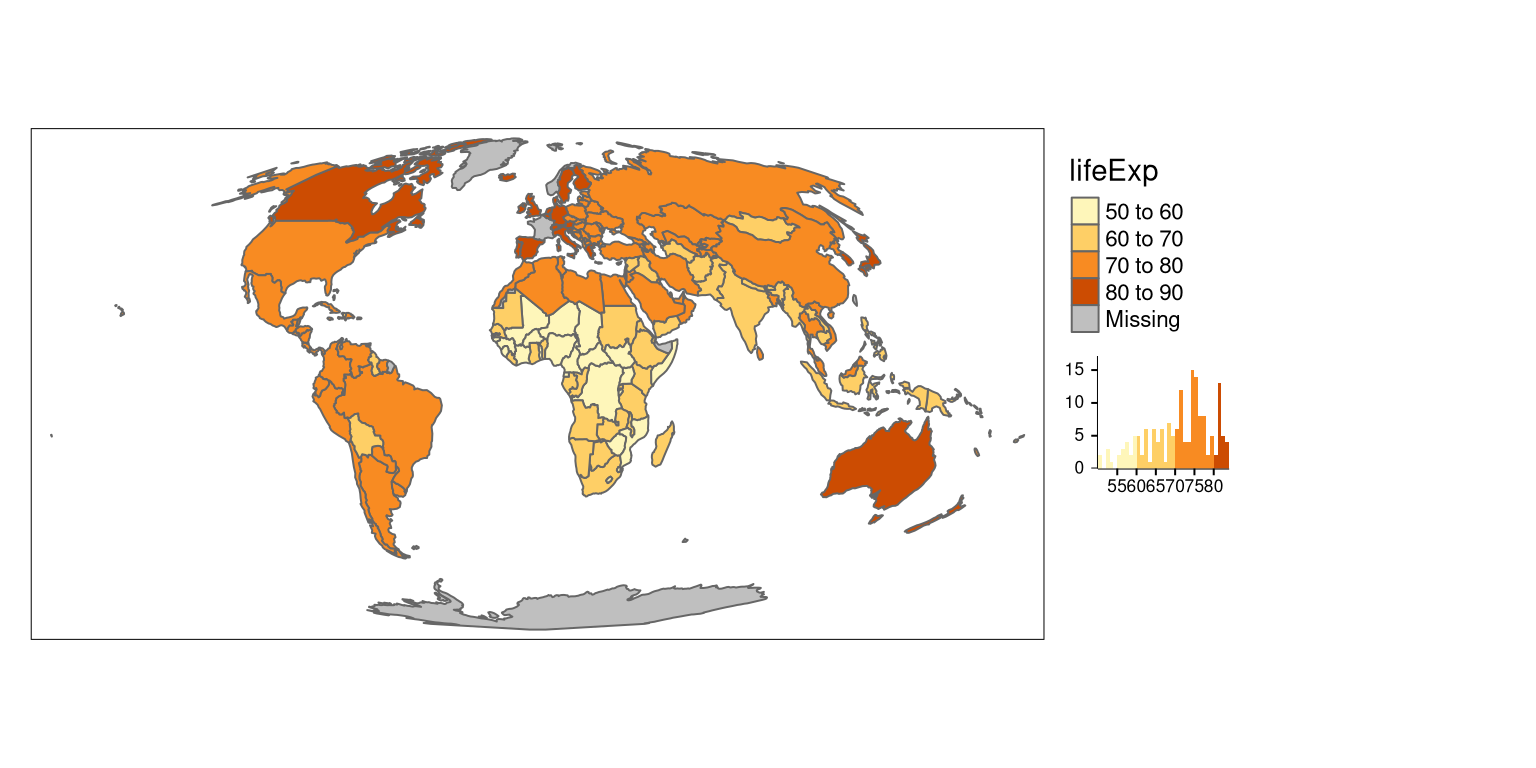## Fixed

The `"fixed"` style allows for a manual selection of the breaks in conjunction with the `breaks` argument.

``````tm_shape(world_moll) +
tm_polygons(col = "lifeExp",
style = "fixed",
breaks = c(45, 60, 75, 90),
legend.hist = TRUE) +
tm_layout(legend.outside = TRUE) ``````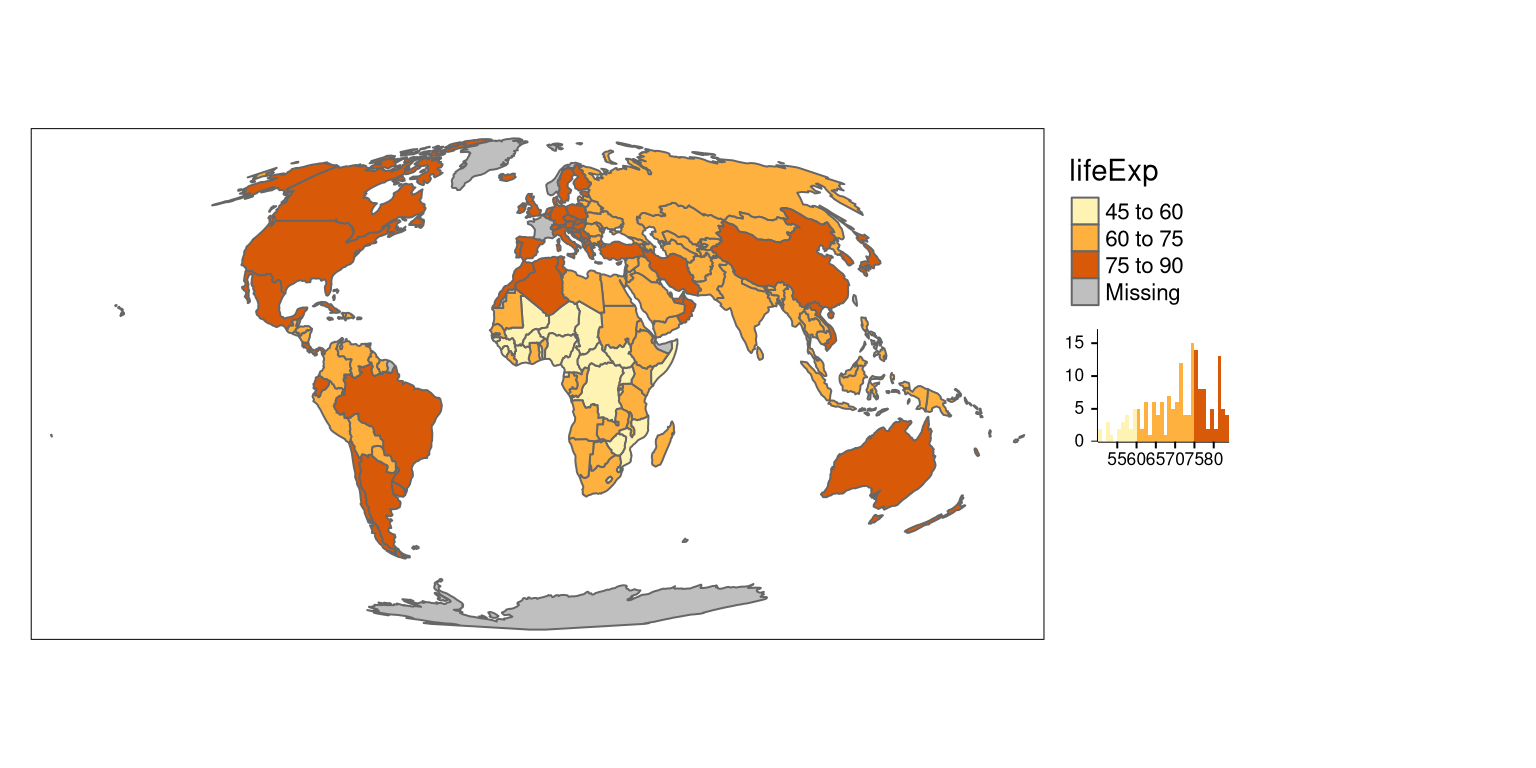Additionally, the default labels can be overwritten using the `labels` argument.

``````tm_shape(world_moll) +
tm_polygons(col = "lifeExp",
style = "fixed",
breaks = c(45, 60, 75, 90),
labels = c("low", "medium", "high"),
legend.hist = TRUE) +
tm_layout(legend.outside = TRUE) ``````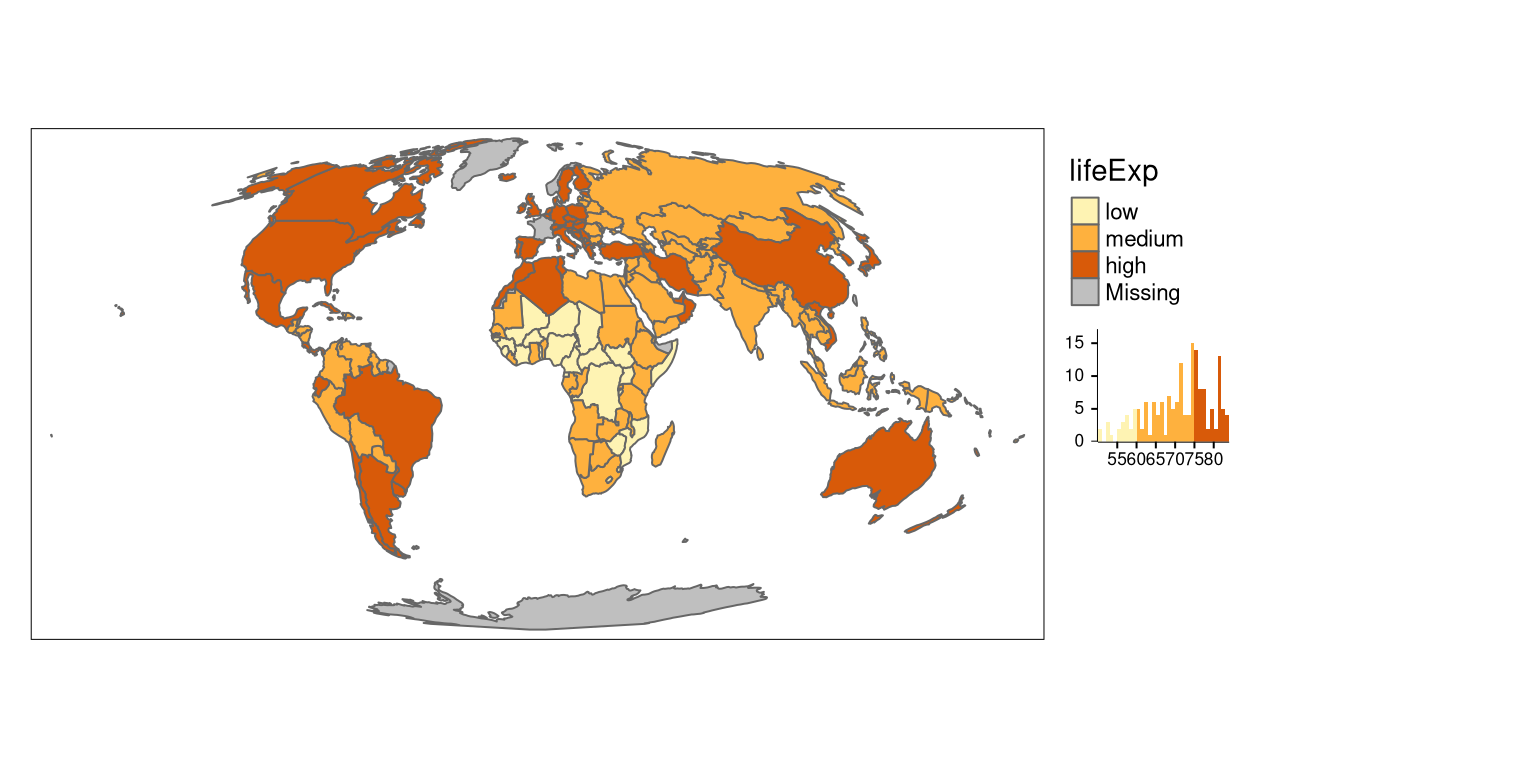## Breaks based on the standard deviation value

The `"sd"` style calculates a standard deviation of a given variable, and next use this value as the break width.

``````tm_shape(world_moll) +
tm_polygons(col = "lifeExp",
style = "sd",
legend.hist = TRUE) +
tm_layout(legend.outside = TRUE)``````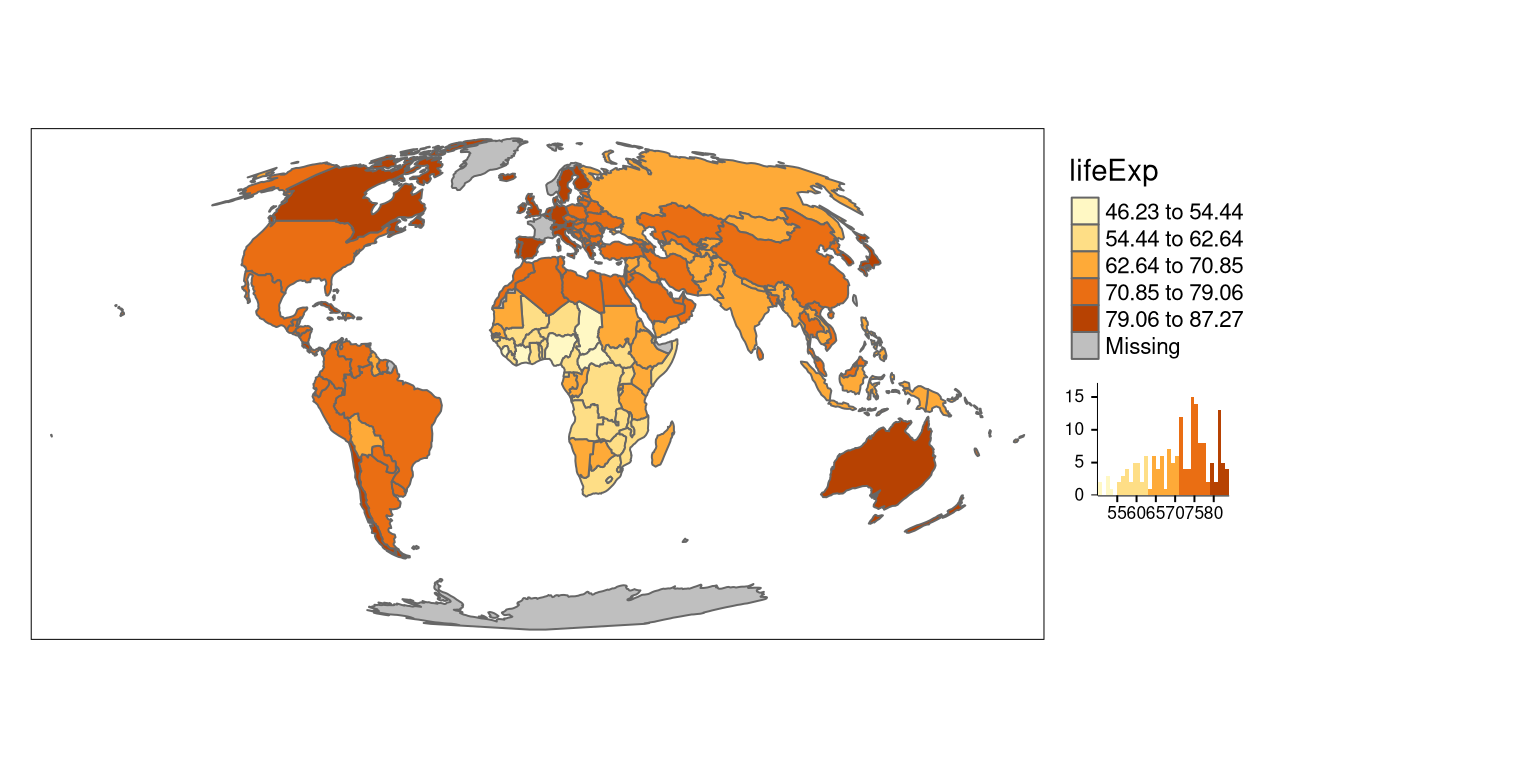## Fisher algorithm

The `"fisher"` style creates groups with maximalized homogeneity.1

``````tm_shape(world_moll) +
tm_polygons(col = "lifeExp",
style = "fisher",
legend.hist = TRUE) +
tm_layout(legend.outside = TRUE) ``````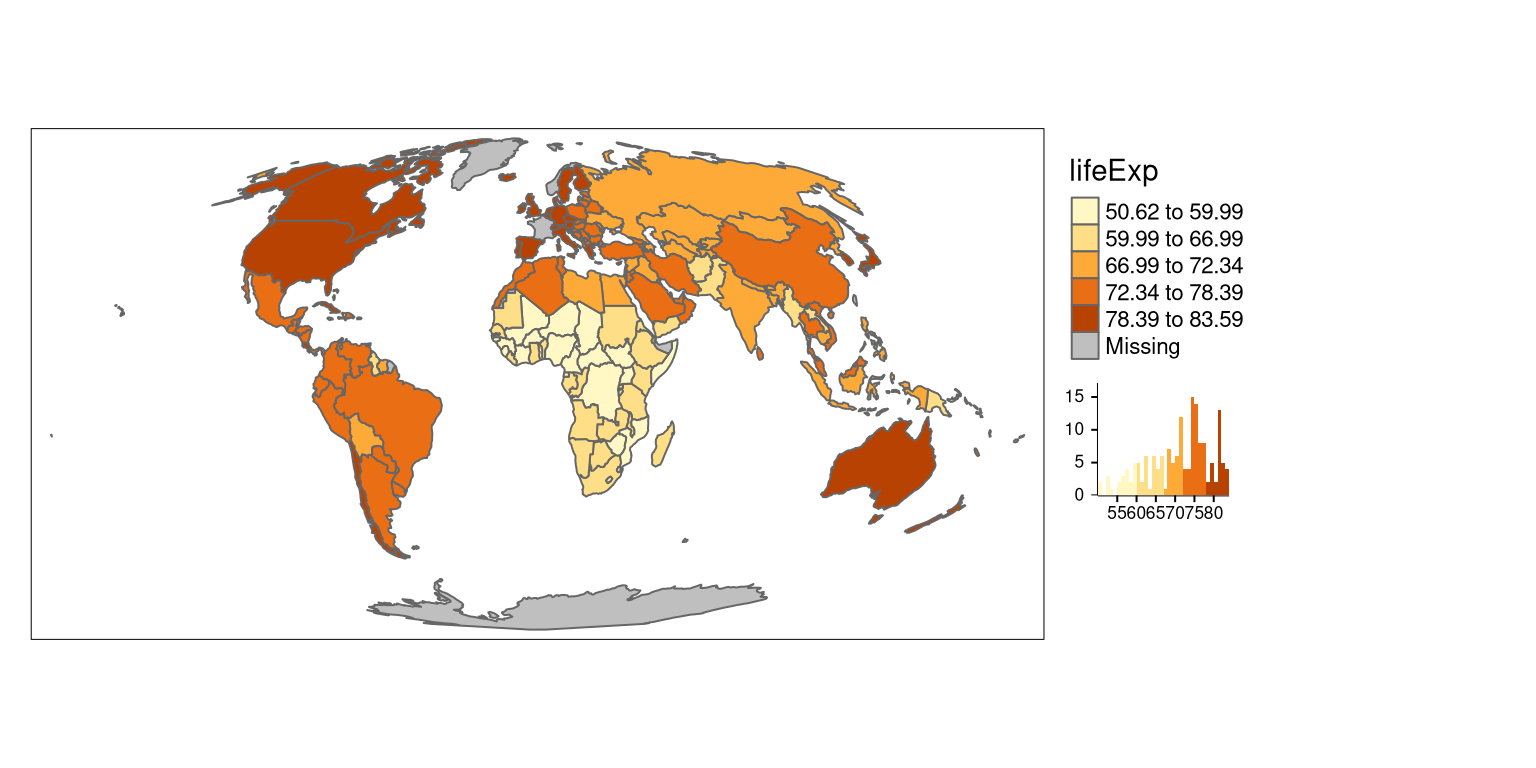## Jenks natural breaks

The `"jenks"` style identifies groups of similar values in the data and maximizes the differences between categories.2

``````tm_shape(world_moll) +
tm_polygons(col = "lifeExp",
style = "jenks",
legend.hist = TRUE) +
tm_layout(legend.outside = TRUE) ``````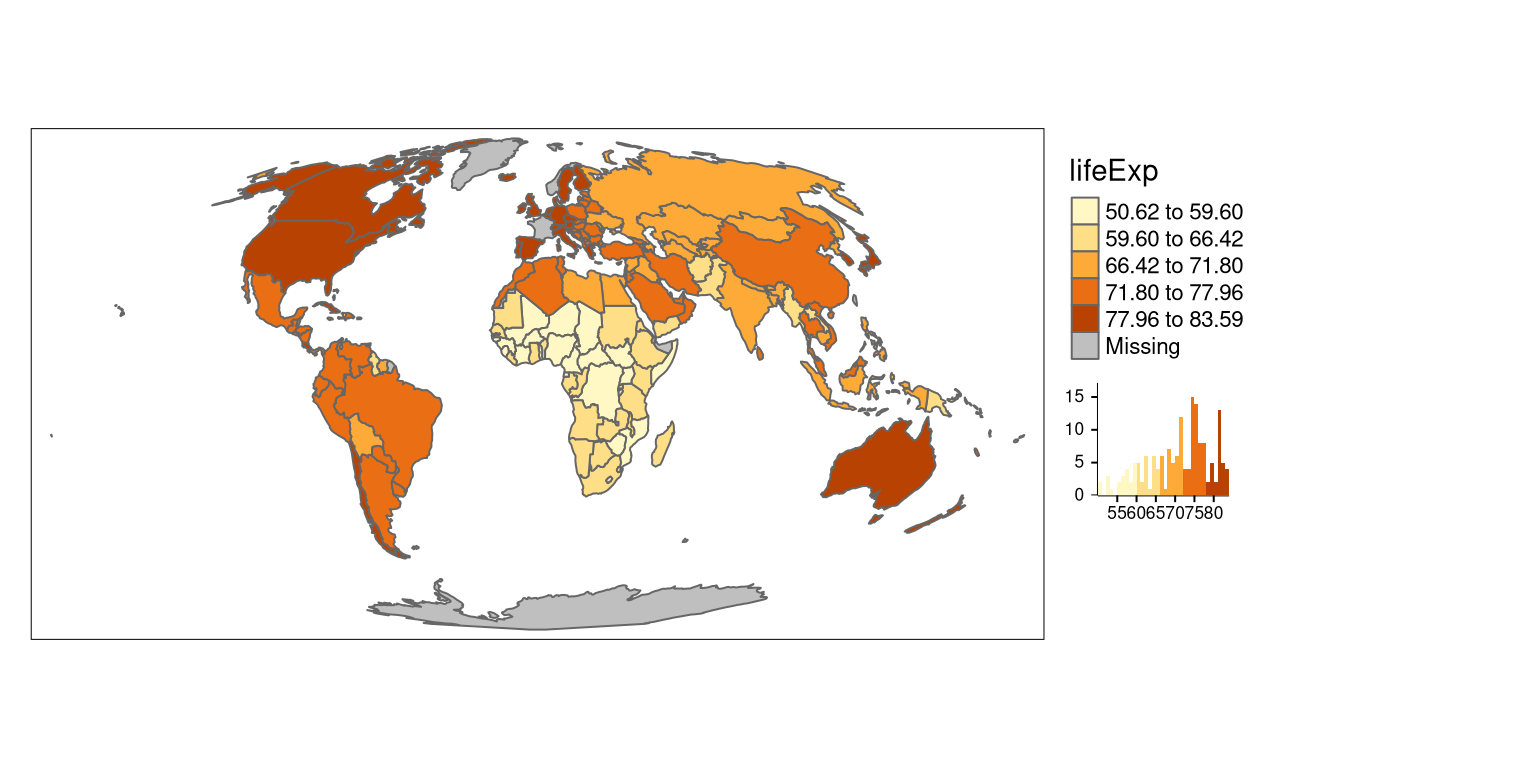## Hierarchical clustering

In the `"hclust"` style, breaks are created using hierarchical clustering.3

``````tm_shape(world_moll) +
tm_polygons(col = "lifeExp",
style = "hclust",
legend.hist = TRUE) +
tm_layout(legend.outside = TRUE) ``````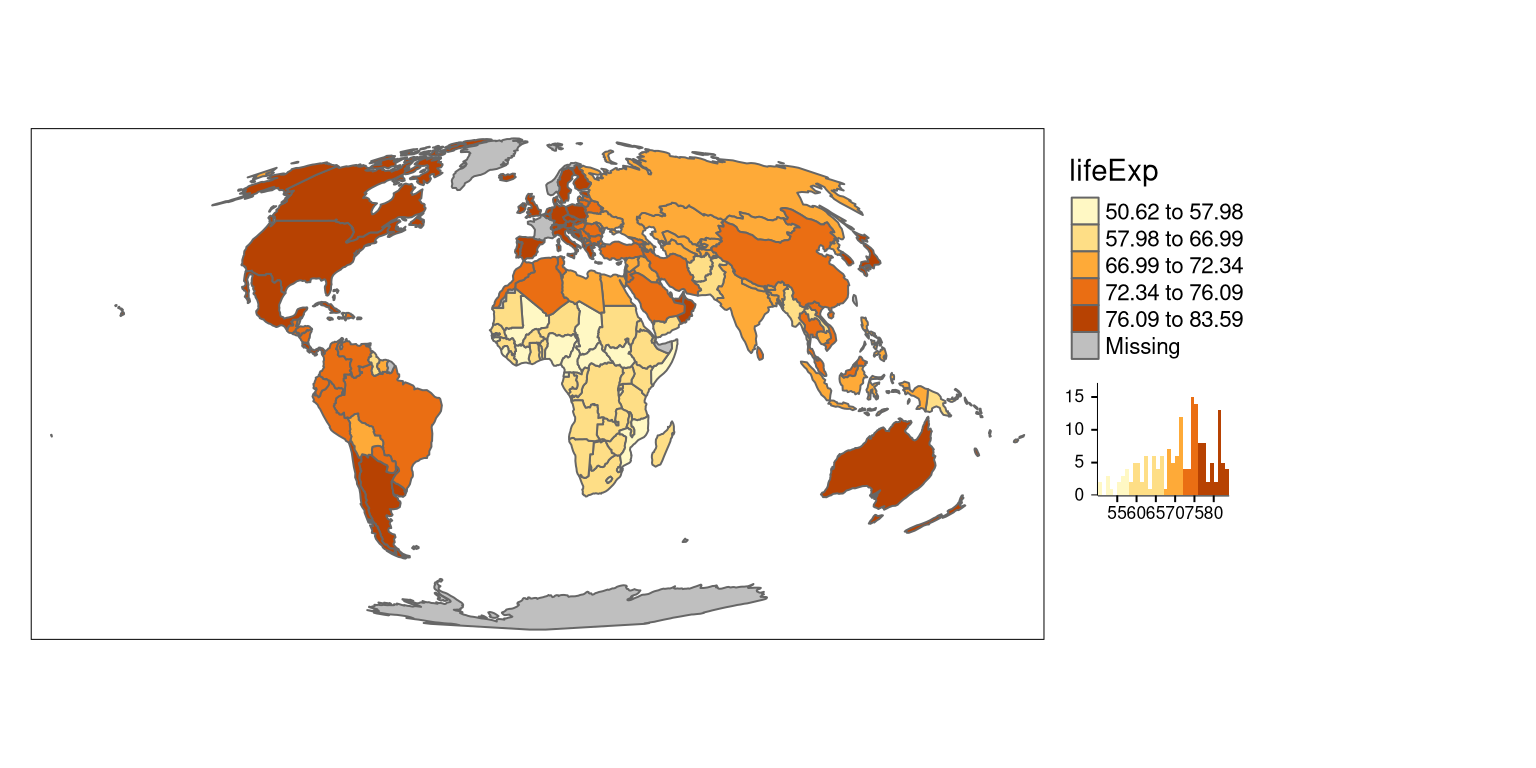## Bagged clustering

The `"bclust"` style uses the `bclust` function to generate the breaks using bagged clustering.4

``````tm_shape(world_moll) +
tm_polygons(col = "lifeExp",
style = "bclust",
legend.hist = TRUE) +
tm_layout(legend.outside = TRUE) ``````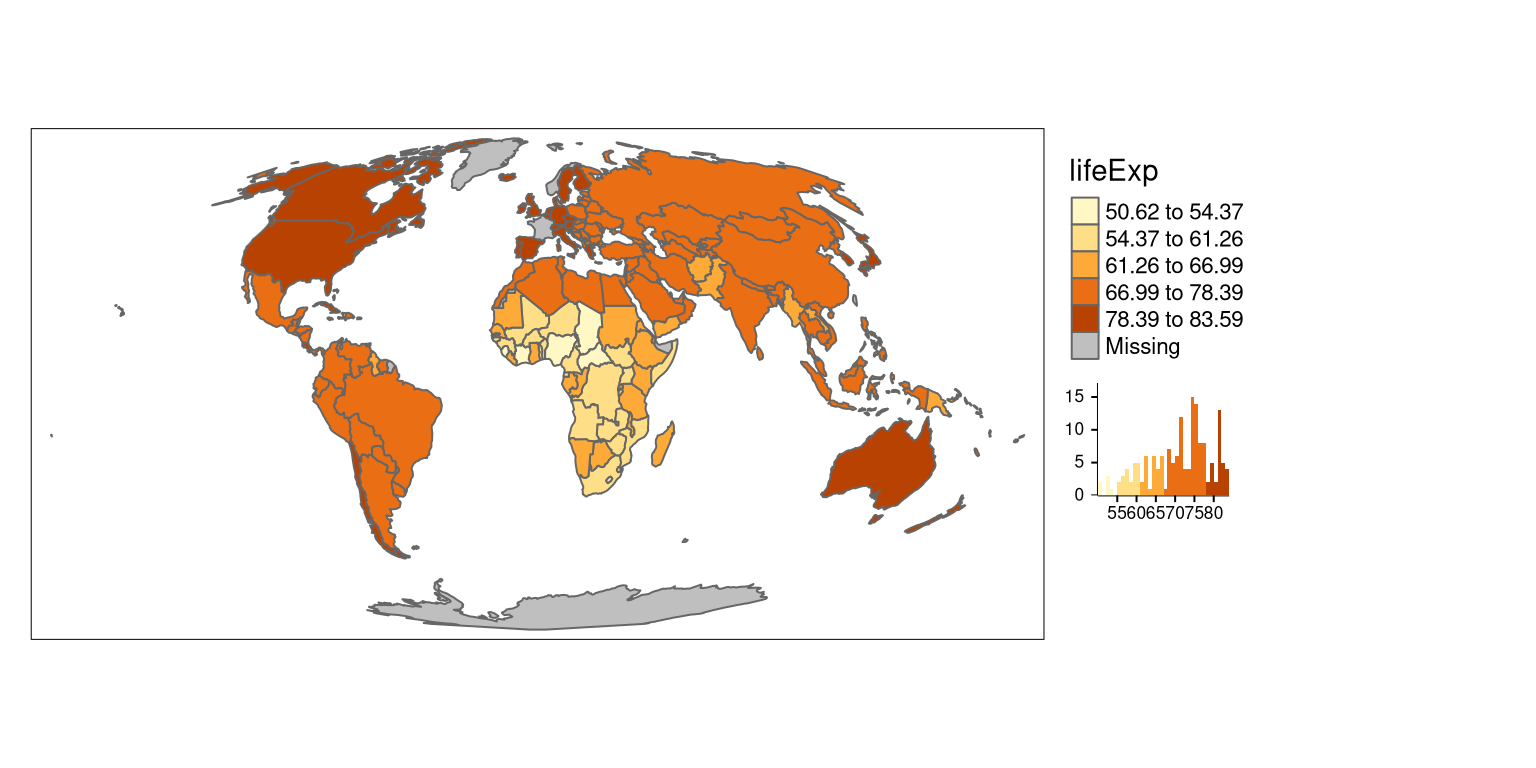``````Committee Member: 1(1) 2(1) 3(1) 4(1) 5(1) 6(1) 7(1) 8(1) 9(1) 10(1)
Computing Hierarchical Clustering``````

## k-means clustering

The `"kmeans"` style uses the `kmeans` function to generate the breaks.5

``````tm_shape(world_moll) +
tm_polygons(col = "lifeExp",
style = "kmeans",
legend.hist = TRUE) +
tm_layout(legend.outside = TRUE) ``````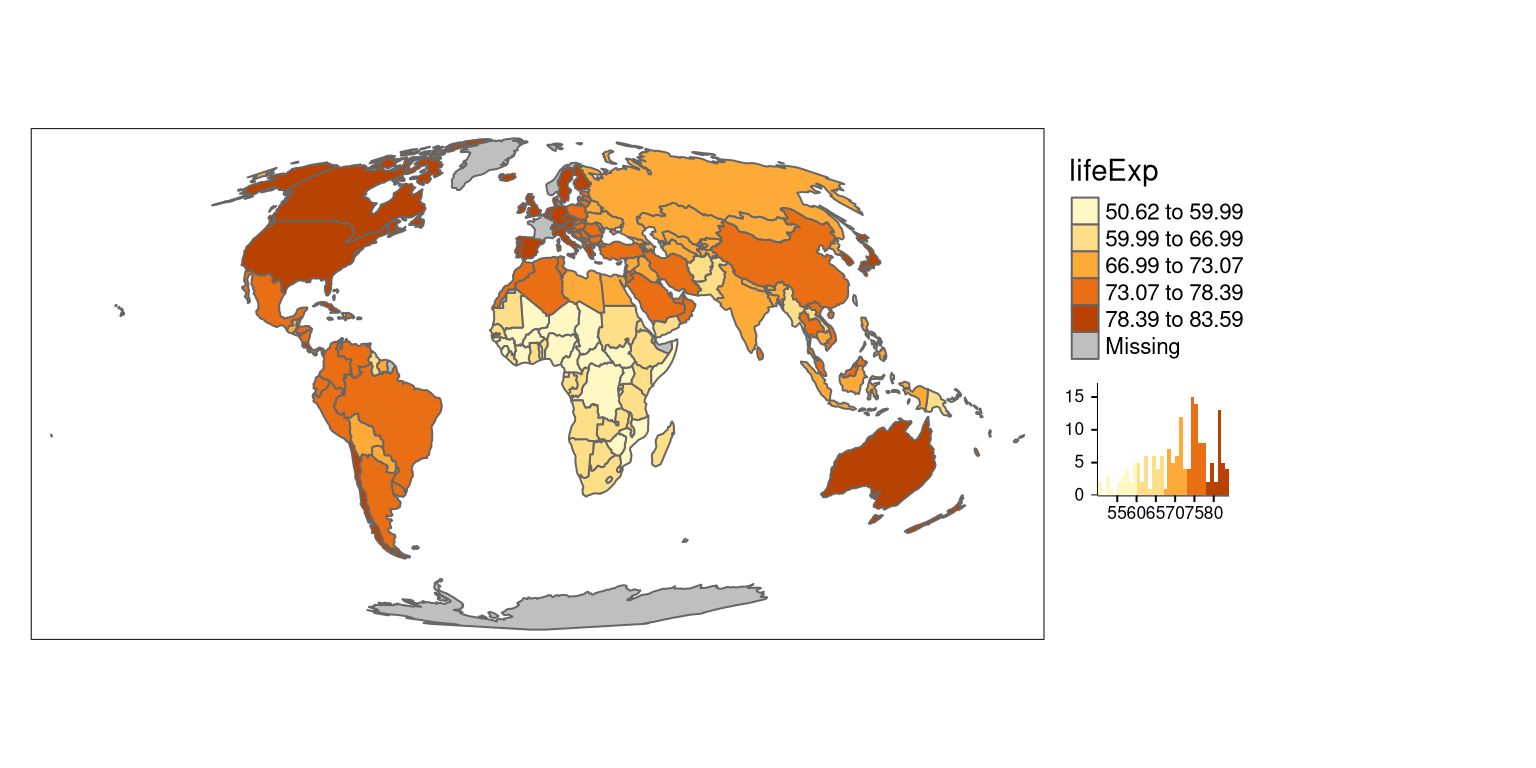## Quantile breaks

The `"quantile"` style creates breaks with an equal number of features (polygons).

``````tm_shape(world_moll) +
tm_polygons(col = "lifeExp",
style = "quantile",
legend.hist = TRUE) +
tm_layout(legend.outside = TRUE) ``````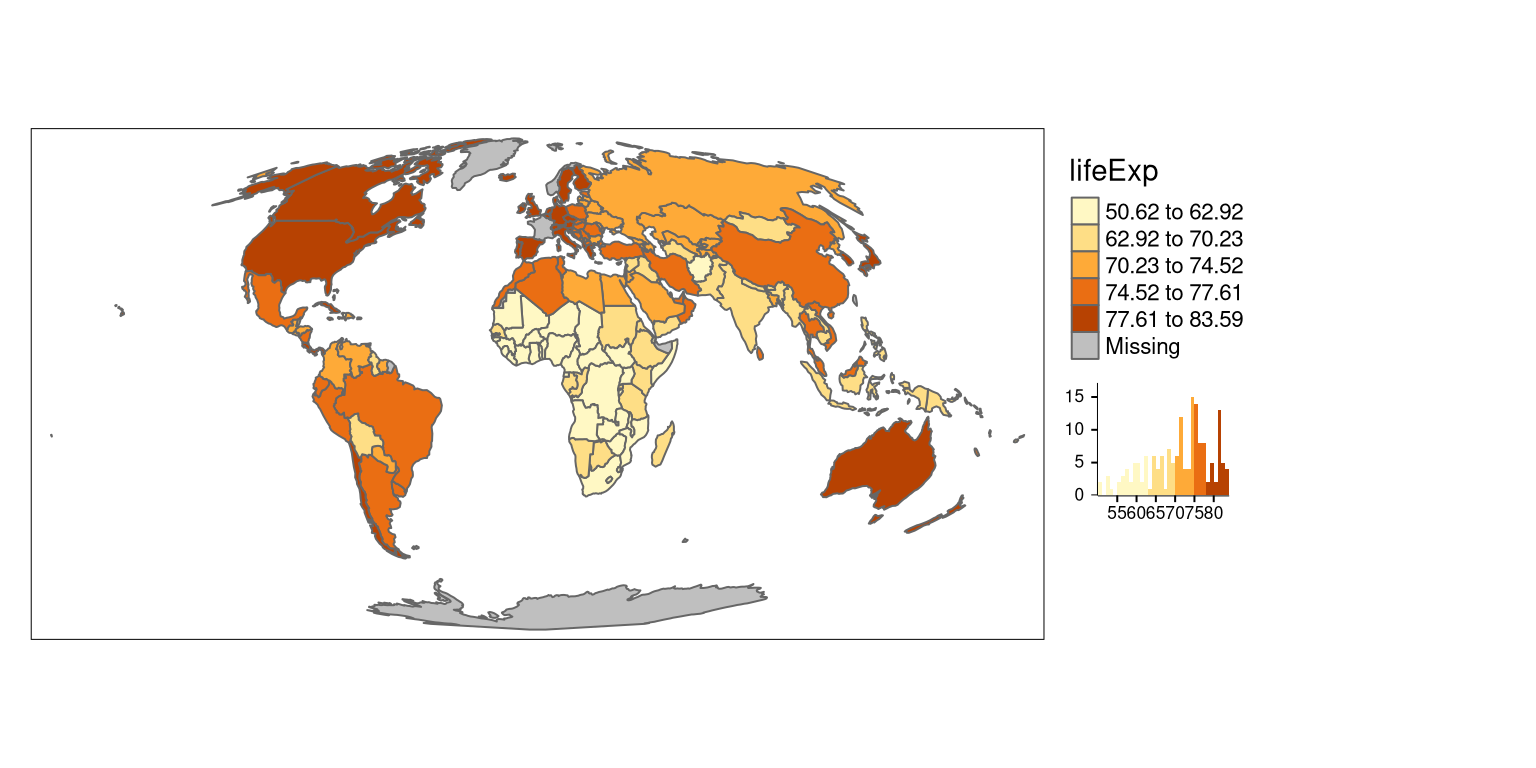## Equal breaks

The `"equal"` style divides input values into bins of equal range and is appropriate for variables with a uniform distribution. It is not recommended for variables with a skewed distribution as the resulting map may end-up having little color diversity.

``````tm_shape(world_moll) +
tm_polygons(col = "lifeExp",
style = "equal",
legend.hist = TRUE) +
tm_layout(legend.outside = TRUE) ``````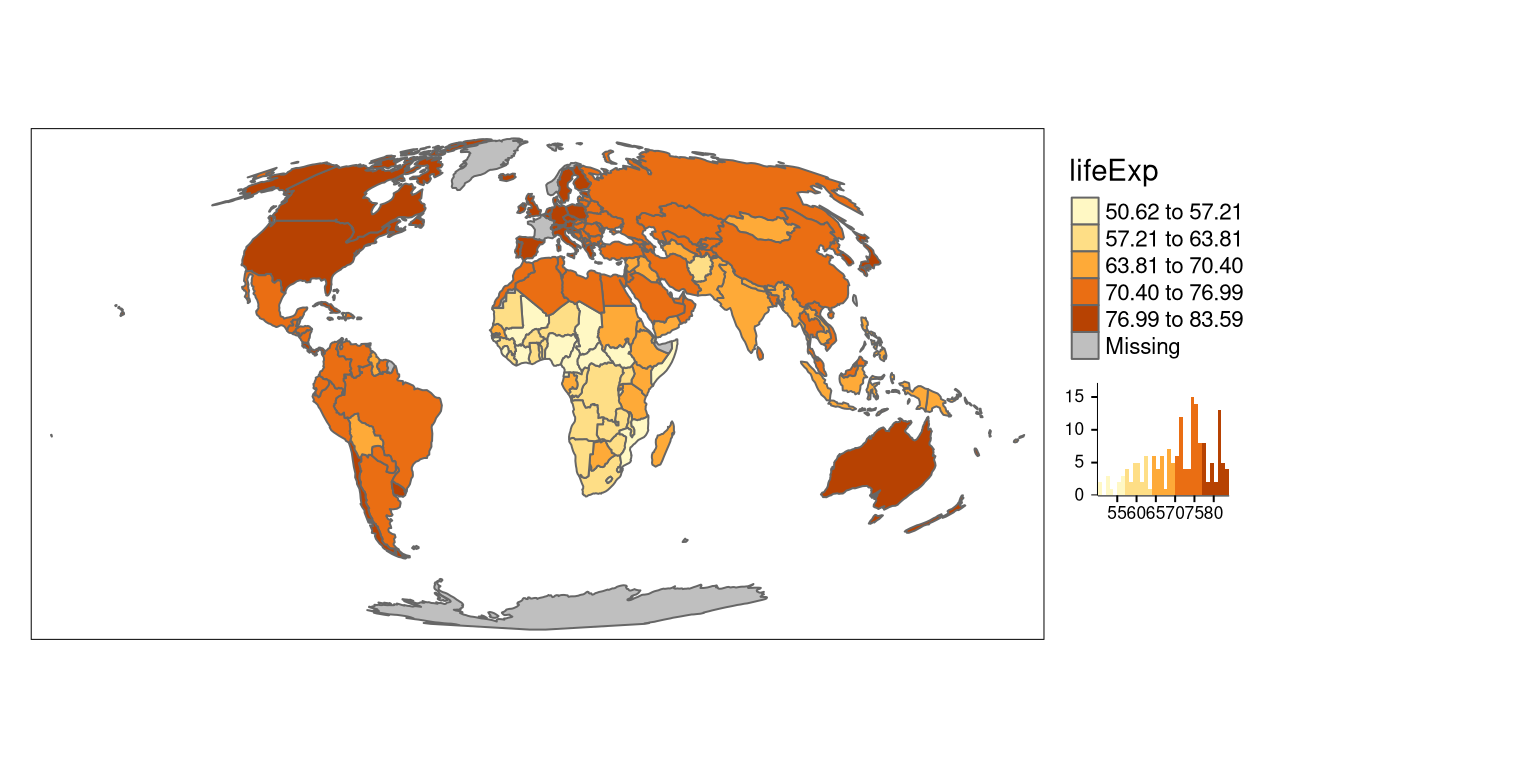# Continuous maps

The tmap package also allows for creating continuous maps.

## Continuous

The `"cont"` style presents a large number of colors over the continuous color field.

``````tm_shape(world_moll) +
tm_polygons(col = "lifeExp",
style = "cont") +
tm_layout(legend.outside = TRUE) ``````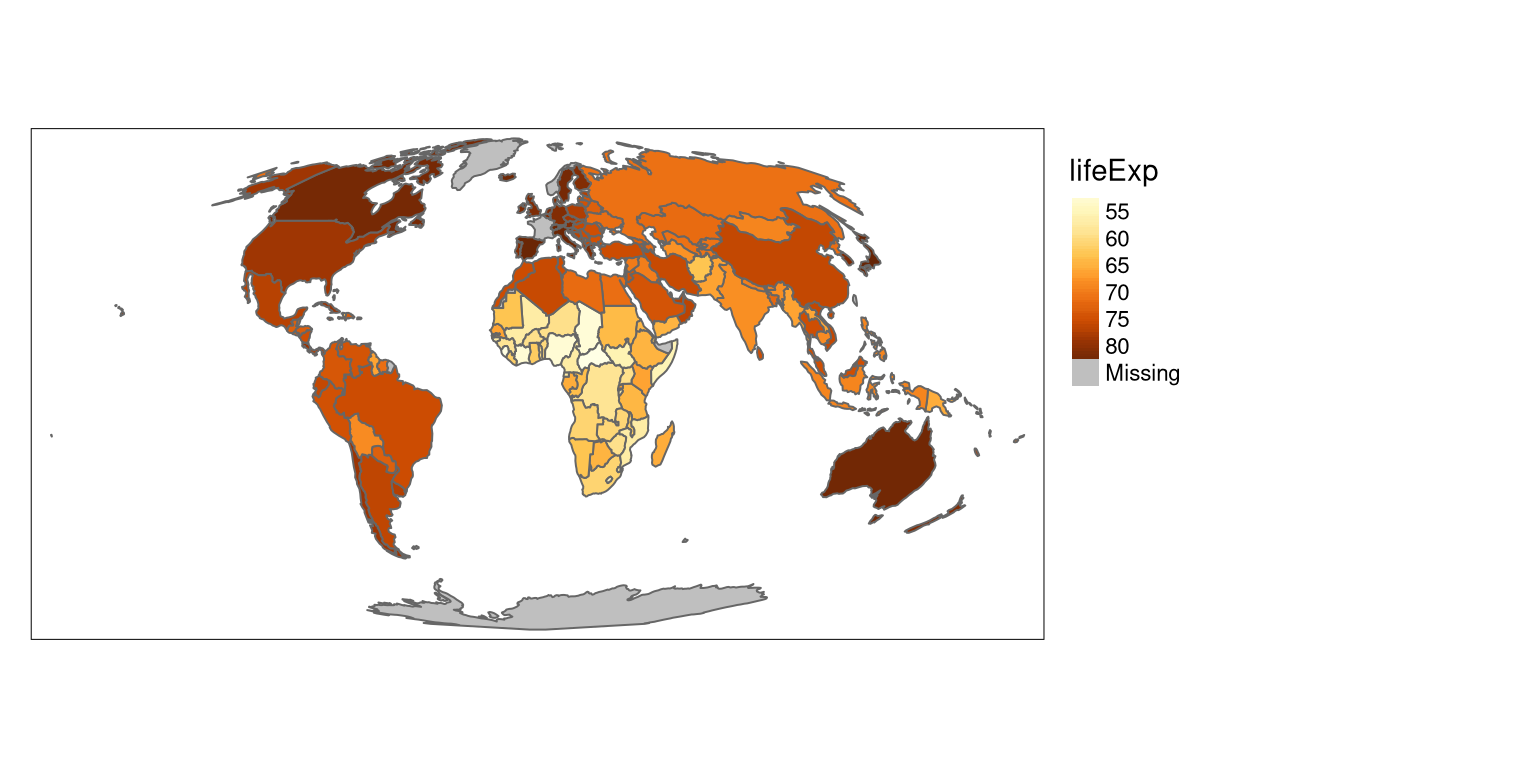## Order

The `"order"` style also presents a large number of colors over the continuous color field. However, this style is suited to visualize skewed distributions; notice that the values on the legend do not change linearly.

``````tm_shape(world_moll) +
tm_polygons(col = "lifeExp",
style = "order") +
tm_layout(legend.outside = TRUE) ``````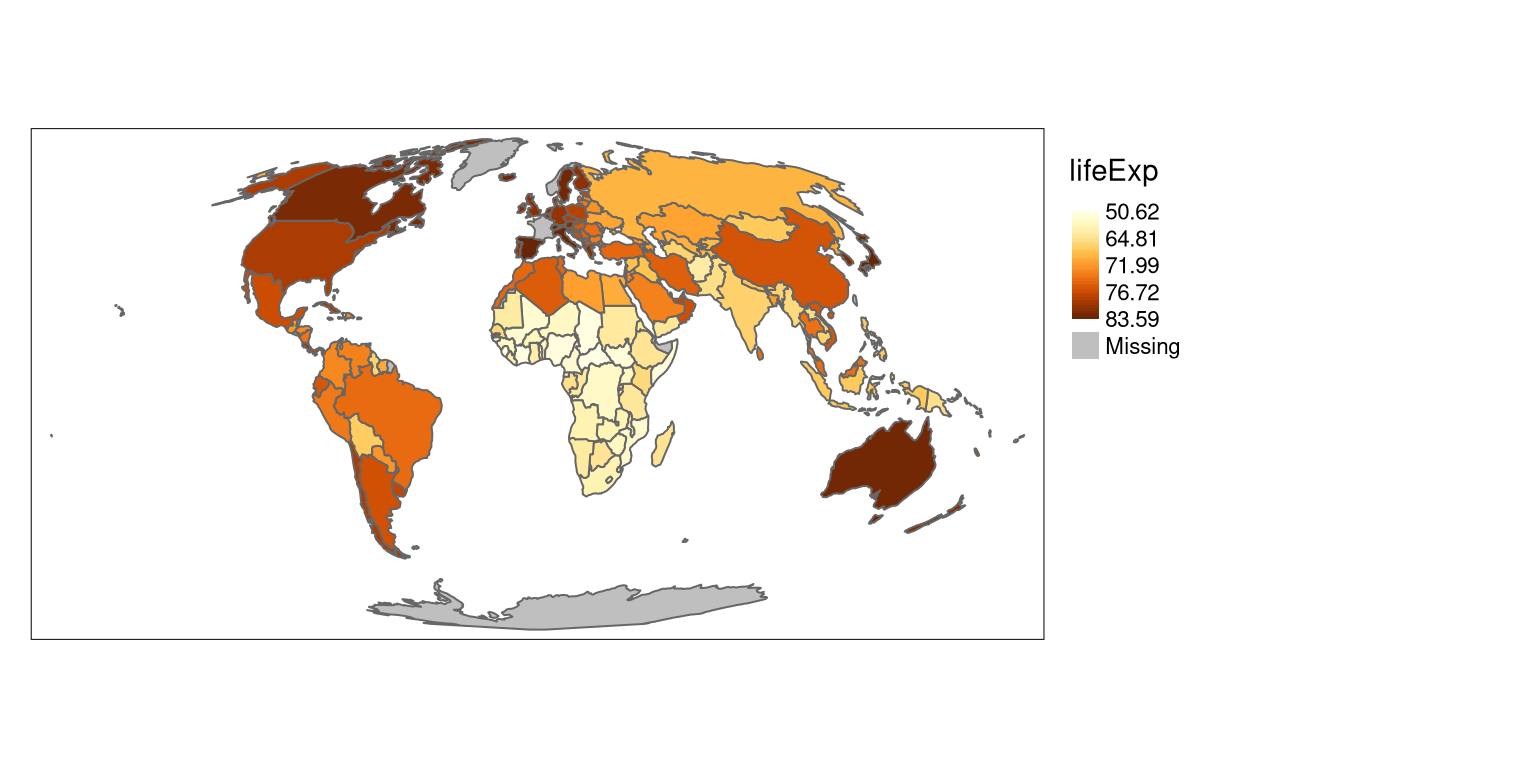# Logarithmic scales

The default numeric style, `pretty`, is easy to understand, but it is not proper for maps of variables with skewed distributions.

``````tm_shape(world_moll) +
tm_polygons(col = "pop") +
tm_layout(legend.outside = TRUE) ``````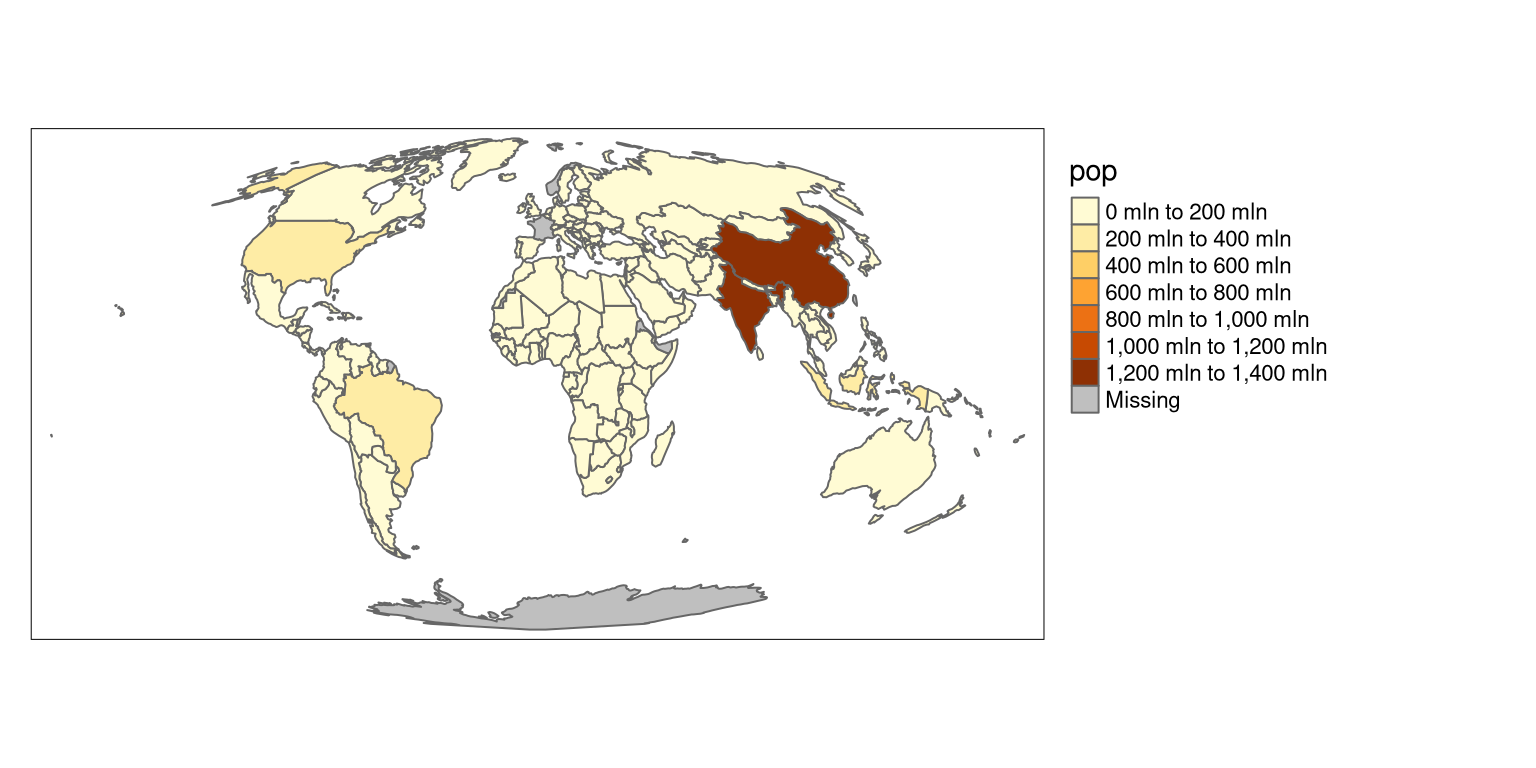Another possible style, `order` works better in this case; however, it is not easy to interpret.

``````tm_shape(world_moll) +
tm_polygons(col = "pop",
style = "order") +
tm_layout(legend.outside = TRUE) ``````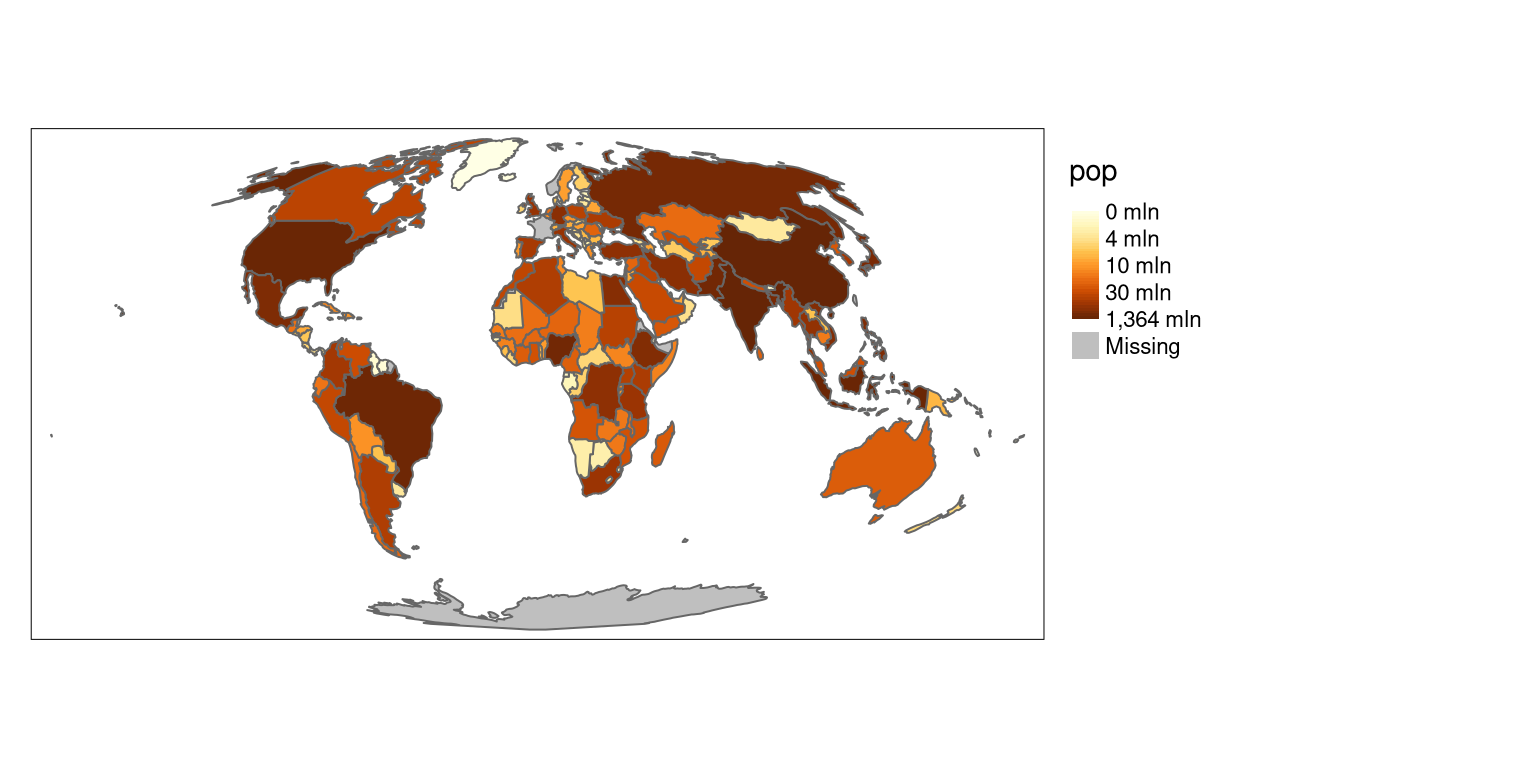A better alternative, in this case, is to use a common logarithm (the logarithm to base 10) scale. The tmap package gives two possibilities in this case - `"log10_pretty"` and `"log10"`. The `"log10_pretty"` style is a common logarithmic version of the regular `pretty` style.

``````tm_shape(world_moll) +
tm_polygons(col = "pop",
style = "log10_pretty") +
tm_layout(legend.outside = TRUE) ``````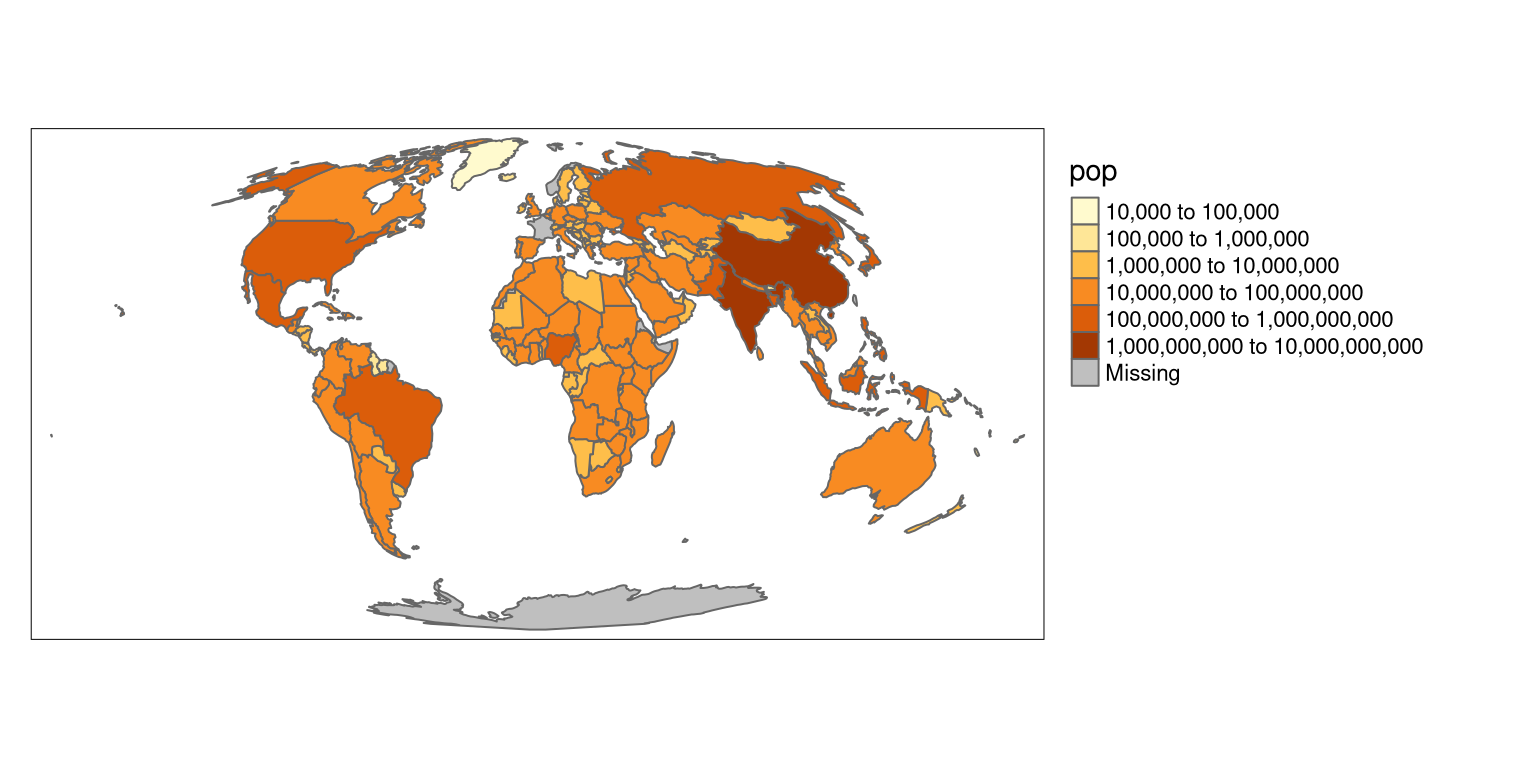On the other hand, the `"log10"` style is a version of a continuous scale.

``````tm_shape(world_moll) +
tm_polygons(col = "pop",
style = "log10") +
tm_layout(legend.outside = TRUE) ``````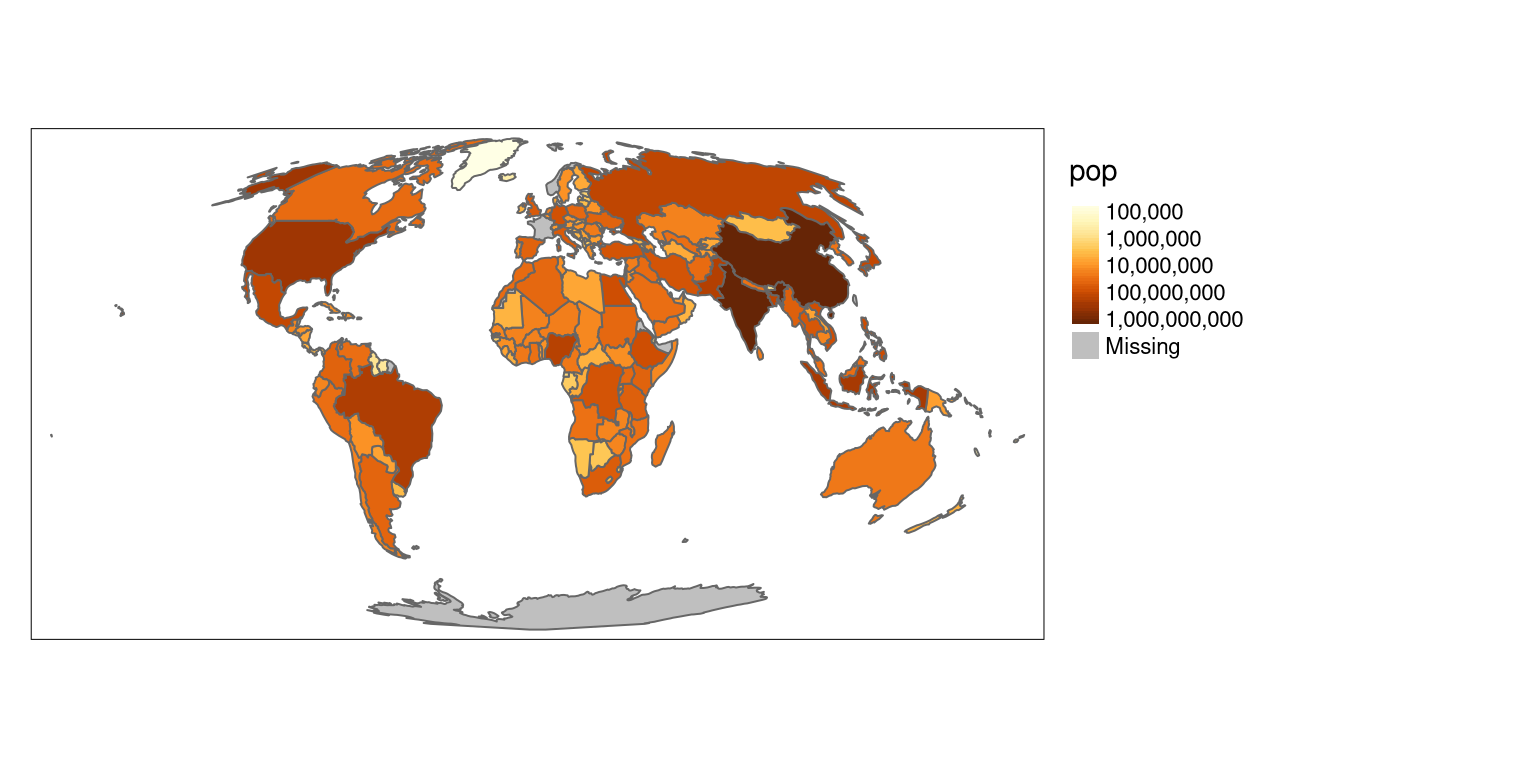# Conclusions

Selecting a color scale style is not an easy task. It depends on the type of input variable and its distribution, but also the intended audience. Therefore, it is worth to spend some time and think about your readers (e.g., would they be able to understand the logarithmic scale or should you use the manual breaks instead?) and your data (e.g., how many breaks should there be to show different subgroups?). Now you know different color scale styles implemented in tmap, so let’s try using them for your own projects!

## Footnotes

1. https://www.tandfonline.com/doi/abs/10.1080/01621459.1958.10501479↩︎

2. https://en.wikipedia.org/wiki/Jenks_natural_breaks_optimization↩︎

3. See the `?hclust` documentation for more details.↩︎

4. See the `?bclust` documentation for more details.↩︎

5. See the `?kmeans` documentation for more details.↩︎

## Citation

BibTeX citation:
``````@online{nowosad2019,